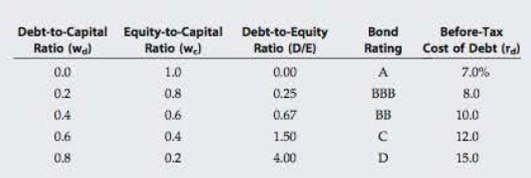Chapter 13, Problem 14SP### Fundamentals of Financial Manageme...

9th Edition
Eugene F. Brigham + 1 other
ISBN: 9781305635937

#### Solutions

Chapter
Section### Fundamentals of Financial Manageme...

9th Edition
Eugene F. Brigham + 1 other
ISBN: 9781305635937
Textbook Problem
14 views

# WACC AND OPTIMAL CAPITAL STRUCTURE Elliott Athletics is trying to determine its optimal capital structure, which now consists of only debt and common equity. The firm does not currently use preferred stock in its capital structure, and it does not plan to do so in the future. Its treasury staff has consulted with investment bankers. On the basis of those discussions, the staff has created the following table showing the firm's debt cost at different debt levels:Elliott uses the CAPM to estimate its cost of common equity, rs, and estimates that the risk-free rate is 5%, the market risk premium is 6%, and its tax rate is 40%. Elliott estimates that if it had no debt, its “unlevered” beta, bU, would be 1.2. a. What is the firm's optimal capital structure, and what would be its WACC at the optimal capital structure? b. If Elliott's managers anticipate that the company's business risk will increase in the future, what effect would this likely have on the firm's target capital structure? c. If Congress were to dramatically increase the corporate tax rate, what effect would this likely have on Elliott's target capital structure? d. Plot a graph of the after-tax cost of debt, the cost of equity, and the WACC versus (1) the debt/capital ratio and (2) the debt/equity ratio.

a.

Summary Introduction

To identify: The optimal capital structure and debt-to-capital to minimize WACC of company.

Optimal Capital Structure:

Optimal capital structure refers a satisfied debt-to-equity ratio through which the cost of capital of a firm can be minimized.

Explanation

Statement to show the calculation of WACC

b.

Summary Introduction

To identify: The effect of increase in company’s business risk.

c.

Summary Introduction

To identify: The effect of increase in tax rate on capital structure of company.

d.

(1)

Summary Introduction

To prepare: The graph of after-tax cost of debt, cost of equity, and WACC versus (1) Debt-to-capital ratio.

(2)

Summary Introduction

To prepare: The graph of after-tax cost of debt, cost of equity, and WACC versus (2) Debt-to-equity ratio.

### Still sussing out bartleby?

Check out a sample textbook solution.

See a sample solution

#### The Solution to Your Study Problems

Bartleby provides explanations to thousands of textbook problems written by our experts, many with advanced degrees!

Get Started